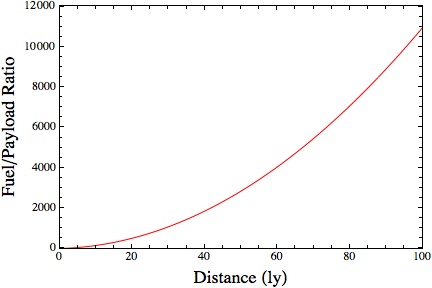# Engineering Problems

In Physics by Brian Koberlein3 Comments

When teaching my physics classes, I’ll often joke when giving examples such as the 1-gee rocket that the details of its construction are merely an engineering problem.  Someone wanted me to post a graph of just how difficult that engineering problem is in this case.

To that end, suppose on our rocket we had a perfectly efficient engine that converted 100% of the fuel into energy for the ship.  This gives us an idea of just how much fuel we would need (at minimum). In the figure below I’ve plotted the ratio of fuel to payload as a function of distance.  You can see that even for a relatively short interstellar trip of 100 light years we need over 10,000 kilograms of fuel for every kilogram of payload.And on that note, I have to get back to grading exams.

1.2.1.Author